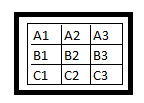## Thursday, December 03, 2015

### Math, Math, Math

Need math?
Dude, no path.
Fills us with wrath
Swells us with wreath
Oh! Not to grave
But to grow.
Math, math, math,
Mother of truth
Author of faith
Dude, it's the path.
In depth of doubt
Math keeps us straight.
Work math at home
Strike with warm chime.

## Thursday, November 12, 2015

### Determining the Determinant of a 3x3 matrix

Introduction
Determinant is a unique value representing a square matrix. It is useful in determining the inverse of a matrix. The determinant of a 1x1 matrix is the entry in the matrix itself whereas in the case of a 2x2 matrix, it is the upper left into lower right minus the upper right into the lower left. The determinant thus obtained is called the minor of the 2x2 matrix. Conventionally, the determinant of a higher order matrix is calculated by a recursive method with the help of the minors. Let us now see a method of rotations to find the determinant.
Let us consider the 2x2 matrix given on the left. Its determinant as per the rule given above is AD-BC.

However, look at the diagram given right. It can be explained as follows.
Rotation Method

Rotate left the second row to one position and then multiply the entries in the respective columns. Subtract the second column product from that of the first.This process can be extended into higher orders also.  This is done by carefully completing all the rotations depending on the number of rows. Let us see the case of a 3x3 matrix.

At first, rotate left the second row to one position and third row to two positions. The matrix thus obtained is given on the right.

Multiply the values in each column separately and then add the three products.
Thus we get A= (A1.B2.C3)+(A2.B3.C1)+(A3.B1.C2).

Now we rotate left the second row to one more position and the third row to two more positions. The matrix thus obtained is as follows.

Multiply the values in each column separately and then add the three products. Thus we get B=(A1.B3.C2)+(A2.B1.C3)+(A3.B2.C1).

The determinant of the 3x3 matrix is then
A-B = (A1.B2.C3+A2.B3.C1+A3.B1.C2)-( A1.B3.C2+A2.B1.C3+A3.B2.C1).

Cylindrical Rotation Method

This process is better understood if we can represent the matrix cylindrically. Consider a cylinder that has three horizontal sections which can be rotated freely with respect to a central vertical axis. Entries in the matrix are given on the exterior of the cylinder.

After the first set of two types of left rotations, the cylinder looks like what is on the left.

Multiplying the entries in the columns separately we get
A=(A1.B2.C3)+(A2.B3.C1)+(A3.B1.C2).

After the second set of two types of left rotations, the cylinder looks like what is on the right.

B = (A1.B3.C2)+(A2.B1.C3)+(A3.B2.C1).

The determinant of the 3x3 matrix is then
A-B = (A1.B2.C3+A2.B3.C1+A3.B1.C2)-( A1.B3.C2+A2.B1.C3+A3.B2.C1).

Palm Method

This can be visualized in yet another way also.
Let us use the three central fingers on the left palm to represent a 3x3 matrix.

Instead of the first set of rotations, multiply the entries from left as indicated by the dark lines, starting from the diagonal to get the value A.

To get the value B, we can multiply the entries from right starting from the antidiagonal. This can be done also by turning the palm and then multiplying the entries from left as given below from the diagonal.

Conclusion

An advantage of the above mentioned process is the elimination of the repeated use of plus(+) and minus(–) which is sometimes disturbing for beginners and non-Mathematics students. Can this method be extended to higher order matrices?

## Monday, September 07, 2015

### Before I became a Teacher

Before I became a Teacher never I knew

That sleeping in the class disturbs
The teaching of the teacher.
That talking in the class makes
The teacher shouting in the class.
That not scribbling in the class augments
That correcting the answer scripts is tougher
Than attempting all the questions.
That questioning the answers is harder
That coming late for the class is worse
Than not attending the class.
That absenting in the class is worse
Than not joining for the course.
That photocopying is the greatest insult
That a teacher can ever bear.
That helping a fellow student learn is
The second greatest insult to a teacher.
That teaching is the noblest profession
When all your students become teachers.

## Saturday, September 05, 2015

### The Teacher I adore and Fear...

First impression is the best
But he proved me wrong, my trust
By being different, still a good teacher
Never roamed behind us like a preacher
Fills my eyes with few tears
As I give my ears when he is sad and cares
And he sends deep in me shivers
When he is angry and brings in my fears
Still he tries his best to put me in front
Although we never succeed, he didn't grit
Waiting, giving us our needed space
Putting us equally with the race
Also makes me admire his knowledge
Which through his words clearly acknowledges
Am sure greater heights are awaiting
To celebrate his humble lovely teaching
He is my teacher whom I adore
But fears put me back far from his doors
Doors of wisdom that is open to all
Spread even if no inner voice gives a call.

On the height of my self-doubt, given to me by one of my students unexpectedly on the Teacher's Day of 2015.

### Develop a skeleton for each day

One has to develop one's own habit of writing. One important  characteristic of habit is that one may not realize what one does in a hab...